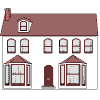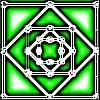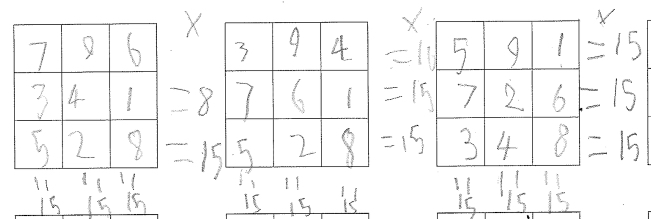#### You may also like### Homes

Six new homes are being built! They can be detached, semi-detached or terraced houses. How many different combinations of these can you find?### Number Squares

Start with four numbers at the corners of a square and put the total of two corners in the middle of that side. Keep going... Can you estimate what the size of the last four numbers will be?### I'm Eight

Find a great variety of ways of asking questions which make 8.

# What's in a Name?

##### Age 5 to 7Challenge Level

Shivangi from the Garden Suburb Junior School”¨ had an interesting idea of choosing what the total for six additions (three across and three down) should be. That did not work so Shrivanga tried splitting her total into groups of three numbers to see if that would produce an answer. Here is what she said:

To solve the problem, I first chose to choose a number for my magic square. Then I decided to break down my number so the numbers would add up to the beginning number. I did not succeed with that solution. Another is that I tried to divide my number equally so they add up to my number. This solution might not work for everyone but it worked for me because my number is 15.

Aleksandra and Hannah from The Glasgow Academy”¨ sent in:

735
249
681

A teacher from Blue Gate Fields Junior School”¨ sent in the following, explaining that the students wrote these comments during the lesson next to their workings out.  They haven't found an answer yet but are continuing to try to solve the problem:

"I am trying to put a big number and then a small number in each row and column to try to get the same total because if I put all the bigger numbers together then the total will be bigger than other rows or columns."

"1+2+3+4+5+6+7+8+9 = 45 and there need to be three digits in each row so I think the totals have to make 15 so I am adding numbers together to make 15 before writing them in the squares."

"I am placing the large numbers at the sides and the small numbers in the middle so they are mixed up."

"I am mixing up the small numbers and the big numbers because if you put all the big numbers together or the small numbers together, you would get different totals."Thank you so much for sending us your thinking. It is really helpful to know how you are going about the problem.

Lily and Harry from Harrison Primary School wrote:
”¨

So when we started we tried to find what number each row needed to add up to so to do that we needed to find the mean and that was 15 so we put 5 in the centre because it was 3 x 5 = 15 and each row had 3. So then we started to put the other numbers around the edge until we got

816
357
492

Then after we got one we just started to move the numbers around to get many different possibilities:

438
951
276

And

294
753
618

And so on we also flipped the numbers around so they where backwards.

Thank you for those solutions. Well done!